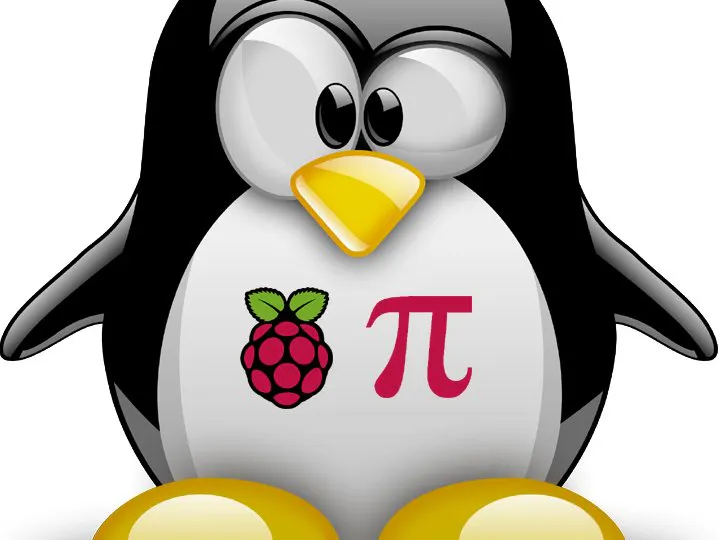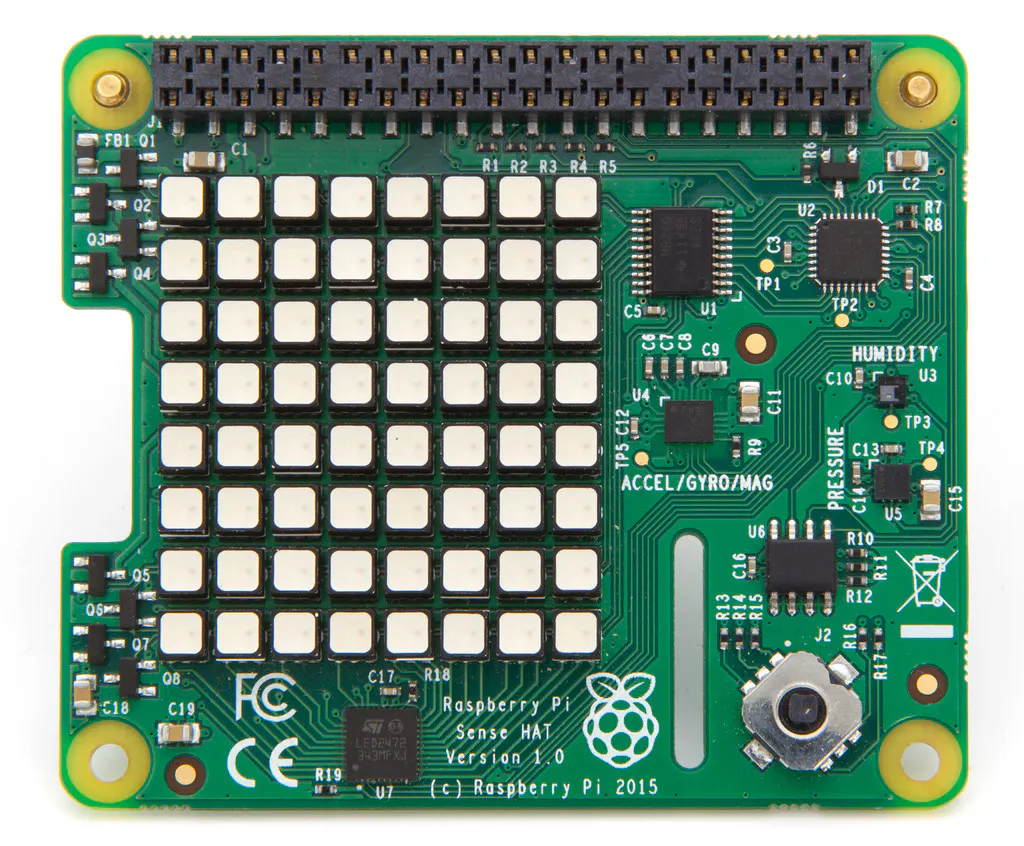Published

# Rpi Sense Hat Clock and Weather Data

It displays time/date, temp in C & F, heat index, dew point, dew/fog, frost warning, humidity, air pressure, delta-t, analog clock and more.

BeginnerShowcase (no instructions)1 hour1,821## Things used in this project

### Hardware componentsRaspberry Pi Sense HAT
×1

## Code

### rpi Sense Hat Weather and clock

Python
```#
# Temperature will take about 5 minutes to settle down as it adjusts for the Pi CPU temp.
# Clock will need an internet connection to sync time
# Joystick change will happen after the current code cycle finishes
# Joystick button = shutdown the Pi
# Joystick up/down = LED brightness
# Joystick Left = scrolling weather
# Joystick Right = clock display
# Create Cron job to start script on Pi boot = @reboot python /home/pi/sensehat.py &
#

import os
import time, datetime
from sense_hat import SenseHat, ACTION_PRESSED, ACTION_HELD, ACTION_RELEASED

sense = SenseHat()
sense.set_rotation(180)
sense.set_imu_config(False, False, False)
sense.low_light = True

s=(0.070) 	# text scroll speed
code=(0)	# which section of code to run

# Dim LED's if joystick Down pressed (Default run state)
def pushed_up(event):
if event.action == ACTION_PRESSED:
sense.low_light = True

# Brighten LED's if joystick Up pressed
def pushed_down(event):
if event.action == ACTION_PRESSED:
sense.low_light = False

# scrolling weather
def pushed_left(event):
if event.action == ACTION_PRESSED:
global code
code = 0

# clock display
def pushed_right(event):
if event.action == ACTION_PRESSED:
global code
code = 1

# shutdown the Pi
def pushed_middle(event):
if event.action == ACTION_PRESSED:
global code
code = 5

sense.stick.direction_up = pushed_up
sense.stick.direction_down = pushed_down
sense.stick.direction_left = pushed_left
sense.stick.direction_right = pushed_right
sense.stick.direction_middle = pushed_middle

# get CPU temperature
def get_cpu_temp():
t = float(res.replace("temp=","").replace("'C\n",""))
return(t)

# use moving average to smooth readings
def get_smooth(x):
if not hasattr(get_smooth, "t"):
get_smooth.t = [x,x,x]
get_smooth.t = get_smooth.t
get_smooth.t = get_smooth.t
get_smooth.t = x
xs = (get_smooth.t+get_smooth.t+get_smooth.t)/3
return(xs)

while True:

# Run code if joystick Right pressed (default code that runs)
if code == 0:

#	# Display the date and time
dateString = "%a %-d%b %-I:%M"
msg = "%s" % (datetime.datetime.now().strftime(dateString))
sense.show_message(msg, scroll_speed=s, text_colour=(0, 255, 255))

# Get senser data
t1 = sense.get_temperature_from_humidity()
t2 = sense.get_temperature_from_pressure()
t_cpu = get_cpu_temp()
h = sense.get_humidity()
p = sense.get_pressure()

# calculates the real temperature compensating for CPU heating
t = (t1+t2)/2
t_corr = t - ((t_cpu-t)/1.5)
t_corr = get_smooth(t_corr)
t_corr = round(t_corr)
t_corr = int(t_corr)		#Celsius
tf = ((t_corr * 9/5) + 32)
tf = round(tf)
tf = int(tf)				#Fahrenheight

# Temperature display
if t_corr <= 5:
tc = [0, 0, 0] # white
elif t_corr > 5 and t_corr < 13:
tc = [0, 0, 255]  # blue
elif t_corr >= 13 and t_corr < 18:
tc = [255, 255, 0]  # yellow
elif t_corr >= 18 and t_corr <= 26:
tc = [0, 255, 0]  # green
elif t_corr > 26:
tc = [255, 0, 0]  # red
msg = "temp %sc (%sf) -" % (t_corr, tf)
sense.show_message(msg, scroll_speed=s, text_colour=tc)

# Calculate the Heat Index
T2 = pow(tf, 2)
H2 = pow(h, 2)
C1 = [ -42.379, 2.04901523, 10.14333127, -0.22475541, -6.83783e-03, -5.481717e-02, 1.22874e-03, 8.5282e-04, -1.99e-06]
heatindex1 = C1 + (C1 * tf) + (C1 * h) + (C1 * tf * h) + (C1 * T2) + (C1 * H2) + (C1 * T2 * h) + (C1 * tf * H2) + (C1 * T2 * H2)
hic1 =  ((heatindex1 - 32) * 5/9)
hic1 = round(hic1)
hic1 = int(hic1)
msg = "feels like %sc -" % (hic1)
sense.show_message(msg, scroll_speed=s, text_colour=tc)

# Calculate the dew point
d = t_corr-(14.55+0.114*t_corr)*(1-(0.01*h))-((2.5+0.007*t_corr)*(1-(0.01*h)))**3-(15.9+0.117*t_corr)*(1-(0.01*h))**14
d = round(d)
d = int(d)

# Calculate the difference between dew point & current temp
e = t_corr - d
e = round(e)
e = int(e)

# Dew point display
if d >= 24:
dp = [255, 0, 0] #red
msg = "dew pt %sc (very humid) -" % (d)
elif d >= 16 and d < 24:
dp = [0, 255, 0] #green
msg = "dew pt %sc (humid) -" % (d)
elif d >= 10 and d < 16:
dp = [0, 255, 0] #Green
msg = "dew pt %sc -" % (d)
elif d < 10:
dp = [255, 255, 0] #yellow
msg = "dew pt %sc (dry) -" % (d)
sense.show_message(msg, scroll_speed=s, text_colour=dp)

# Water Vapour
if e <= 2:
msg = "dew/fog -"
hb = [255, 0, 0]  # red
sense.show_message(msg, scroll_speed=s, text_colour=hb)
elif e > 3 and e <= 6:
msg = "dew/fog possible -"
hb = [255, 255, 0]  # yellow
sense.show_message(msg, scroll_speed=s, text_colour=hb)
else:
msg = "no fog -"
hb = [0, 255, 0]  # green
#		sense.show_message(msg, scroll_speed=s, text_colour=hb)

# Frost point warning
if e <= 2 and t_corr <= 1:
msg = "FROST WARNING -"
fw = [255, 0, 0]  # red
sense.show_message(msg, scroll_speed=s, text_colour=fw)

# Humidity display
h = sense.get_humidity()
h = round(h)
h = int(h)
if h > 100:
h = 100
if h >= 30 and h <= 60:
hc = [0, 255, 0]  # green
msg = "humidty %s%% -" % (h)
sense.show_message(msg, scroll_speed=s, text_colour=hc)
elif h > 60 and h < 80:
hc = [255, 255, 0]  # yellow
msg = "humidty %s%% -" % (h)
sense.show_message(msg, scroll_speed=s, text_colour=hc)
else:
hc = [255, 0, 0]  # red
msg = "humidty %s%% -" % (h)
sense.show_message(msg, scroll_speed=s, text_colour=hc)

# Air pressure display
p = sense.get_pressure()
p = round(p)
p = int(p)
if p >= 960 and p < 990:
pc = [255, 0, 0]  # red
msg = "baro very low %smb (storm) -" % (p)
sense.show_message(msg, scroll_speed=s, text_colour=pc)
elif p >= 990 and p < 1000:
pc = [255, 255, 0]  # yellow
msg = "baro low %smb (rain) -" % (p)
sense.show_message(msg, scroll_speed=s, text_colour=pc)
elif p >= 1000 and p < 1015:
pc = [0, 255, 0]  # green
msg = "baro mid %smb (mainly fine) -" % (p)
sense.show_message(msg, scroll_speed=s, text_colour=pc)
elif p >= 1015 and p < 1030:
pc = [0, 0, 255]  # blue
msg = "baro high %smb (fine) -" % (p)
sense.show_message(msg, scroll_speed=s, text_colour=pc)
elif p >= 1030:
pc = [255, 0, 0]  # red
msg = "baro very high %smb (dry) -" % (p)
sense.show_message(msg, scroll_speed=s, text_colour=pc)

# Wet Bulb Temperature
Tdc = ((t_corr-(14.55+0.114*t_corr)*(1-(0.01*h))-((2.5+0.007*t_corr)*(1-(0.01*h)))**3-(15.9+0.117*t_corr)*(1-(0.01*h))**14))

E = (6.11*10**(7.5*Tdc/(237.7+Tdc)))

WBc = (((0.00066*p)*t_corr)+((4098*E)/((Tdc+237.7)**2)*Tdc))/((0.00066*p)+(4098*E)/((Tdc+237.7)**2))

# Delta-T - If the Delta T is between 2C and 8C, pesticides are more effective
Dt = t_corr - WBc
Dt = round(Dt)
Dt = int(Dt)
msg = "delta-t %sc" % (Dt)
sense.show_message(msg, scroll_speed=s, text_colour=[0, 255, 0])

# Vapor Pressure(Mb)
Vp = (6.11*10**(7.5*((t_corr-(14.55+0.114*t_corr)*(1-(0.01*h))-((2.5+0.007*t_corr)*(1-(0.01*h)))**3-(15.9+0.117*t_corr)*(1-(0.01*h))**14))/(237.7+((t_corr-(14.55+0.114*t_corr)*(1-(0.01*h))-((2.5+0.007*t_corr)*(1-(0.01*h)))**3-(15.9+0.117*t_corr)*(1-(0.01*h))**14)))))
Vp = round(Vp)
Vp = int(Vp)
msg = "vapor pressure: %sMb -" % (Vp)
#		sense.show_message(msg, scroll_speed=s, text_colour=[0, 255, 0])

# Run code if joystick Left pressed
if code == 1 or code == 0:		# Remove 'or code == 0' if you dont want the clock to display
clock = (0)

u = 60.0/29
zero = [[0,0,1,1,0,0],[0,1,0,0,1,0],[0,1,0,0,1,0],[0,1,0,0,1,0],[0,1,0,0,1,0],[0,0,1,1,0,0]]
one=[[0,0,0,1,0,0],[0,0,1,1,0,0],[0,0,0,1,0,0],[0,0,0,1,0,0],[0,0,0,1,0,0],[0,0,1,1,1,0]]
two=[[0,0,1,1,0,0],[0,1,0,0,1,0],[0,0,0,0,1,0],[0,0,0,1,0,0],[0,0,1,0,0,0],[0,1,1,1,1,0]]
three=[[0,0,1,1,0,0],[0,1,0,0,1,0],[0,0,0,1,0,0],[0,0,0,0,1,0],[0,1,0,0,1,0],[0,0,1,1,0,0]]
four=[[0,0,0,0,1,0],[0,0,0,1,1,0],[0,0,1,0,1,0],[0,1,1,1,1,0],[0,0,0,0,1,0],[0,0,0,0,1,0]]
five=[[0,1,1,1,1,0],[0,1,0,0,0,0],[0,1,1,1,0,0],[0,0,0,0,1,0],[0,1,0,0,1,0],[0,0,1,1,0,0]]
six=[[0,0,1,1,0,0],[0,1,0,0,0,0],[0,1,1,1,0,0],[0,1,0,0,1,0],[0,1,0,0,1,0],[0,0,1,1,0,0]]
seven=[[0,1,1,1,1,0],[0,0,0,0,1,0],[0,0,0,1,0,0],[0,0,0,1,0,0],[0,0,1,0,0,0],[0,0,1,0,0,0]]
eight=[[0,0,1,1,0,0],[0,1,0,0,1,0],[0,0,1,1,0,0],[0,1,0,0,1,0],[0,1,0,0,1,0],[0,0,1,1,0,0]]
nine=[[0,0,1,1,0,0],[0,1,0,0,1,0],[0,1,0,0,1,0],[0,0,1,1,1,0],[0,0,0,0,1,0],[0,0,1,1,0,0]]
ten=[[0,1,0,0,1,0],[1,1,0,1,0,1],[0,1,0,1,0,1],[0,1,0,1,0,1],[0,1,0,1,0,1],[0,1,0,0,1,0]]
eleven=[[0,1,0,0,1,0],[1,1,0,1,1,0],[0,1,0,0,1,0],[0,1,0,0,1,0],[0,1,0,0,1,0],[0,1,0,0,1,0]]
twelve=[[0,1,0,0,1,0],[1,1,0,1,0,1],[0,1,0,0,0,1],[0,1,0,0,1,0],[0,1,0,1,0,0],[0,1,0,1,1,1]]

nums = [zero, one, two, three, four, five, six, seven, eight, nine, ten, eleven, twelve]

# hour background color
z = [0,0,0]

# hour number color
w = [0,0,255]

# minute background color
e = [0,0,0]

# minute dot color
x = [0,150,0]

def row_one(m):
result = [0,0,0,0,0,0,0,0]
if m > u*4 and m < u*25:
result = [0,0,0,0,1,1,1,1]
else:
if m < u:
result = [0,0,0,0,0,0,0,0]
elif m < u*2:
result = [0,0,0,0,1,0,0,0]
elif m < u*3:
result = [0,0,0,0,1,1,0,0]
elif m < u*4:
result = [0,0,0,0,1,1,1,0]
elif m < u*25:
result = [0,0,0,0,1,1,1,1]
elif m < u*26:
result = [1,0,0,0,1,1,1,1]
elif m < u*27:
result = [1,1,0,0,1,1,1,1]
elif m < u*28:
result = [1,1,1,0,1,1,1,1]
else:
result = [1,1,1,1,1,1,1,1]
return result

def row_eight(m):
result = [1,1,1,1,1,1,1,1]
if m < u*18:
if m < u*11:
result = [0,0,0,0,0,0,0,0]
elif m < u*12:
result = [0,0,0,0,0,0,0,1]
elif m < u*13:
result = [0,0,0,0,0,0,1,1]
elif m < u*14:
result = [0,0,0,0,0,1,1,1]
elif m < u*15:
result = [0,0,0,0,1,1,1,1]
elif m < u*16:
result = [0,0,0,1,1,1,1,1]
elif m < u*17:
result = [0,0,1,1,1,1,1,1]
else:
result = [0,1,1,1,1,1,1,1]
return result

def convert_to_color(arr, background, color):
return [background if j == 0 else color for j in arr]

def update_clock(hh, mm):
if hh > 12:
hh = hh - 12

img = []

# row 1
row1 = row_one(mm)
img.extend(convert_to_color(row1, e, x))

# row 2-7
for i in range(6):
if mm > (u*(24-i)):
img.append(x)
else:
img.append(e)

# hour number
img.extend(convert_to_color(nums[hh][i], z, w))

if mm > (u*(5+i)):
img.append(x)
else:
img.append(e)

#row 8
row8 = row_eight(mm)
img.extend(convert_to_color(row8, e, x))
sense.set_pixels(img)

if code == 1:
now = datetime.datetime.now()
update_clock(now.hour, now.minute*1.0)
time.sleep(10)
if code == 0:
while clock < 30:		# will display sesnor data every 5 min if set to 30
now = datetime.datetime.now()
update_clock(now.hour, now.minute*1.0)
time.sleep(10)
clock = clock + 1

# Shutdown the Pi if joystick center button pressed
if code == 5:
sense.show_message("Shutdown", scroll_speed=s, text_colour=[255, 0, 0])
os.system("sudo shutdown now -P")

# Code mangled together by MarkD - Version 1.0 - Dec17
#
# Code Sources:
# Kerry Waterfield - https://forums.pimoroni.com/t/my-portable-weather-clock-astro-pi/5132
# Michael Bell - https://iridl.ldeo.columbia.edu/dochelp/QA/Basic/dewpoint.html